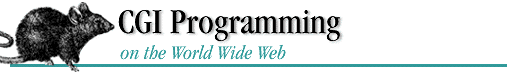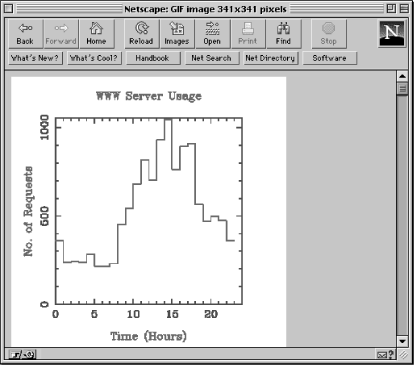#Chapter 6Hypermedia Documents## 6.5 CGI Examples with pgperl

gnuplot is concise and fun for throwing up a few charts, but for sophisticated plotting you may want a more powerful package called pgperl. This is a derivative of Perl that supports the PGPLOT FORTRAN plotting library. Typically it has been used to plot astronomical data, but you can use it to graph any type of data.

You can get pgperl from http://www.ast.cam.ac.uk/~kgb/pgperl.html.

What does pgperl offer that gnuplot doesn't? pgperl contains many powerful plotting functions (all beginning with the prefix "pg"), such as a variety of histograms and mapped contours, which gnuplot doesn't have. Another important consideration is that the pgperl graphic routines are incorporated straight into Perl, and thus there is no need to work with temporary files or pipes. Let's take a look at a pgperl example that graphs the information in the NCSA server log file.

### Web Server Accesses

Here is a pgperl program that is similar in functionality to the gnuplot example above. It is intended to show you the differences between gnuplot and pgperl.

```#!/usr/local/bin/pgperl
require "pgplot.pl";
\$webmaster = "shishir\@bu\.edu";
\$access_log = "/usr/local/bin/httpd_1.4.2/logs/access_log";
```

The require command includes the pgperl header file that consists of various PGPLOT functions.

```\$hours = 23;
\$maximum = 0;
```

The \$maximum variable represents the maximum y coordinate when we plot the histogram. It sets the range on the y axis.

```\$process_id = \$\$;
\$output_gif = join ("", "/tmp/", \$process_id, ".gif");
```

The output_gif variable is used to store the name of a temporary file that will contain the GIF image.

```if ( (open(FILE, "<" . \$access_log)) ) {
for (\$loop=0; \$loop <= \$hours; \$loop++) {
\$time[\$loop] = 0;
\$counter[\$loop] = \$loop;
}
```

Two arrays are initialized to hold the hour and access data. The @time array holds the number of accesses for each hour, and the @counter array represents the hours (0--23).

```    while (<FILE>){
if (m|\[\d+/\w+/\d+:([^:]+)|) {
\$time[\$1]++;
}
}
```

A regular expression identical to the one presented in the last example is used to determine the number of accesses for each hour.

```    close (FILE);
&find_maximum();
&prepare_graph();
} else {
&return_error (500, "Server Log File Error", "Cannot open NCSA server access log!");
}
exit(0);
```

The find_maximum subroutine determines the maximum y value--or the hour that had the most accesses. And the prepare_graph subroutine calls the various pgperl routines to graph the data.

```sub find_maximum
{
for (\$loop=0; \$loop <= \$hours; \$loop++) {
if (\$time[\$loop] > \$maximum) {
\$maximum = \$time[\$loop];
}
}
\$maximum += 10;
}
```

Initially, the maximum value is set to zero. The number of accesses for each hour is checked against the current maximum value to determine the absolute maximum. Finally, the maximum value is incremented by 10 so the histogram doesn't look cramped. In other words, the range on the y axis will be 10 greater than the maximum value that falls on the axis.

```sub prepare_graph
{
&pgbegin (0, "\${output_gif}/VGIF", 1, 1);
&pgscr (0, 1, 1, 1);
```

The pgbegin function creates a portrait GIF image with a black background and stores it in the file specified by \$output_gif. The first argument is reserved for future use, and is currently ignored. The third and fourth arguments specify the number of graphs that should fit horizontally and vertically, respectively, in the image. Finally, the pgscr function remaps a color index. In this case, we are remapping color zero (black) to one (white). Unfortunately, this is the only way to change the background color.

```&pgpap (4.0, 1.0);
```

pgpap is used to change the width and aspect ratio (width / height) of the image. Normally, the image size is 8.5 x 11 inches in portrait mode. An aspect ratio is the ratio between the x axis and the y axis; 1.0 produces a square image. For example, an aspect ratio of 0.618 results in a horizontal rectangle, and a ratio of 1.618 results in a vertical rectangle. This function changes the width to four inches and the aspect ratio to one.

```&pgscf (2);
&pgslw (3);
&pgsch (1.6);
```

The pgscf function modifies the font style to Roman. Here is a list of all the styles:

Style

Attribute

1

Normal

2

Roman

3

Italic

4

Script

The line width and the character height are changed with the pgslw and pgsch functions, respectively.

```&pgsci (4);
&pgenv (0, \$hours + 1, 0, \$maximum, 2, 0);
```

The pgsci function changes the pen color to blue. We use the pgenv function to draw our axes. The range for the x axis goes from 0 to (\$hours + 1), and the range for the y axis is from 0 to the maximum number of accesses plus 10. The fifth argument is responsible for independently scaling the x and y axes. A value of one is used to set equal scales for the x and y axes; any other values cause pgperl to independently scale the axes. The last argument controls the plotting of axes and tick marks. A value of zero instructs pgperl to draw a box around the graph, and to label the coordinates.

```    &pgsci (2);
&pgbin (\$hours, *counter, *time, 0);
&pglabel ("Time (Hours)", "No. of Requests", "WWW Server Usage");
&pgend;
```

The pen color is again modified to two (red). The crucial routine here is pgbin. It draws a histogram with 23 values (represented by \$hours). The x coordinates are specified by the counter array, and the y coordinates--or the number of accesses--are stored in the time array. Notice how the arrays are passed to the pgbin function; they are passed as references--this is a requirement of pgperl. The last argument instructs pgperl to draw the histogram with the edge of each box located at the corresponding x coordinate.

```&print_gif();
}
```

The print_gif subroutine prints the GIF image to standard output.

```sub print_gif
{
local (\$content_length);
if ( (open (GIF, "<" . \$output_gif)) ) {
\$content_length = (stat (GIF));
print "Content-type: image/gif", "\n";
print "Content-length: ", \$content_length, "\n\n";
print <GIF>;
close (GIF);
} else {
&return_error (500, "Server Log File Error",
"Cannot read from the GIF file!");
}
}
```

Notice that we use the Content-length header in this subroutine. Whenever you are returning binary data (such as GIF images) and it is possible to determine the size of the image, you should make it a habit to send this header. The stat command returns the file size of the graphic image. The file is printed to standard output, and deleted. If you like, you can use the algorithm in Chapter 3 to return the GIF image in small pieces.

Figure 6.6 shows the image created by this script.

#### Figure 6.6: pgperl graph